# SSAT Upper Level Math : How to find fractional percentages

## Example Questions

### Example Question #1 : How To Find Fractional Percentages

What percent of 12,000 is 3,300?

28.5%

27.5%

24.5%

26%

25%

27.5%

Explanation:

To find out what percent a partis of a whole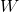, evaluate the expression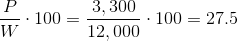### Example Question #2 : How To Find Fractional Percentages

Convert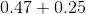into a percent.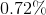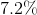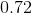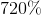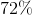Explanation:

First evaluate.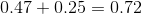To convert a decimal to a percentage, multiply by 100 percent.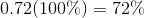### Example Question #3 : How To Find Fractional Percentages

Which of the following best represents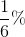?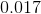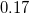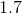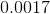Explanation:

The true value of the percentagecan be converted by dividing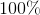.

The correct value ofis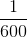.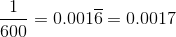### Example Question #4 : How To Find Fractional Percentages

Convert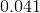into a percent.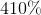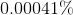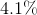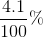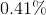Explanation:

To convert a decimal number into a percent, multiply the decimal number by.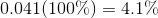### Example Question #5 : How To Find Fractional Percentages

Find the sum of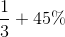.  Express the final answer as a fraction.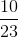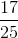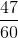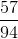Explanation:

Convert the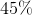into fraction form by dividing this by.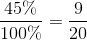First find the common denominator by multiplying the current denominators together.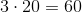Now convert each fraction into an equivalent fraction with the new, common denominator.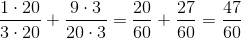### Example Question #6 : How To Find Fractional Percentages

What percentage of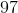is? Round to the nearest hundredth.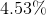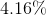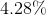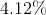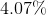Explanation:

Set up a mathematical equation such that the percentage of the number is divided by 100. Solve for the unknown value.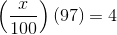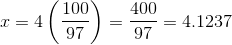The correct answer is.

### Example Question #7 : How To Find Fractional Percentages

Each of a set of balls is marked with a letter of the English alphabet. Each vowel is represented by five balls; each consonant, three. What percent of the balls are marked with vowels? (Nearest whole percent)

Note: For purposes of this problem, "Y" is considered a consonant.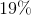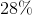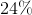Explanation:

There are five vowels, each represented by five balls; this is a total of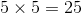balls with vowels.

There are twenty-one consonants, each represented by three balls; this is a total of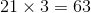balls with consonants.

There are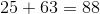balls total of which 25 out of 88 are marked with vowels. This represents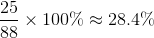of the balls, so 28% is the correct choice.